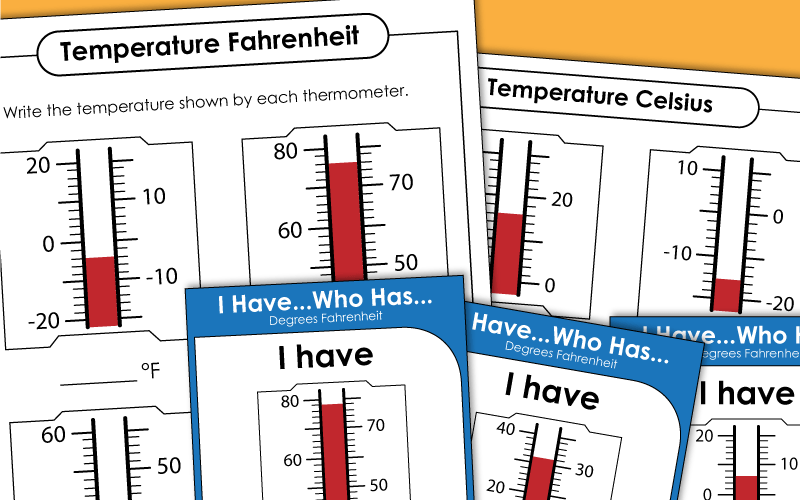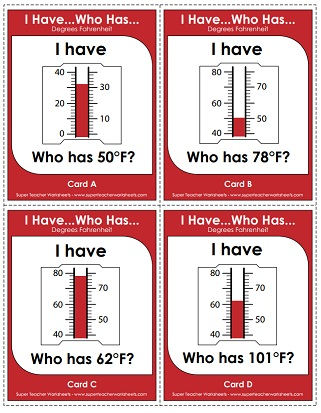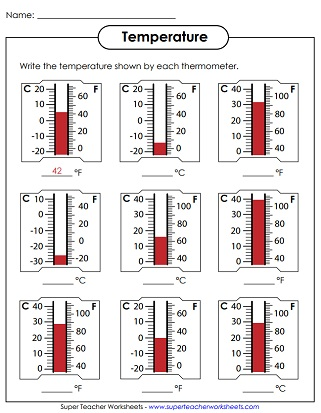# Temperature Measurement

Measure the temperature on the thermometers to the nearest degree in Celsius and Fahrenheit. The units on thermometers are clear and easy-to-read.## Fahrenheit

Write the Fahrenheit temperature shown on each thermometer (Nearest 10 degrees)
Write the temperature shown by each of the 9 thermometers.
Write the Fahrenheit temperature shown on each thermometer (Nearest degree)
Draw the given temperature on each Fahrenheit thermometer.
Practice reading thermometers with this whole-class chain reaction game. All thermometers have Fahrenheit temperatures.

## Celsius

Write the Celsius temperature shown on each thermometer (Nearest 10 degrees)
Write the Celsius temperature shown on each thermometer (Nearest degree)
Draw the given temperature on each Celsius thermometer.
Practice reading thermometers with this whole-class chain reaction game. All thermometers have Celsius temperatures.

## Fahrenheit & Celsius

Cut the labels (water freezes, water boils, room temperature, etc) and attach to the thermometer illustration.
Write the temperature shown on each thermometer. Each thermometer includes Fahrenheit and Celsius units.

Capacity Measurement (Gallons, Quarts)

Use the picture of GallonBot to answer the questions about gallons, quarts, pints, and cups.

Weather Worksheets

Worksheets on temperature, predicting weather, types of weather, and more.

## Worksheet ImagesMy Account
Site Information